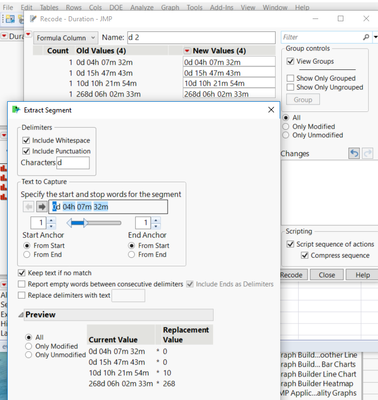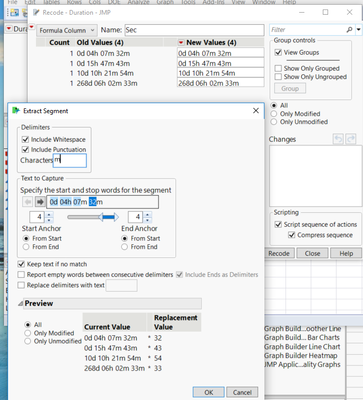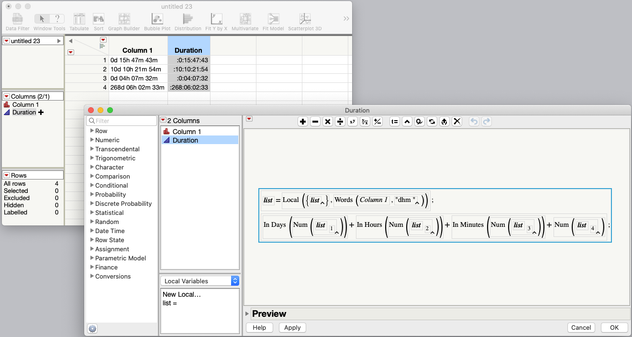Our World Statistics Day conversations have been a great reminder of how much statistics can inform our lives. Do you have an example of how statistics has made a difference in your life? Share your story with the Community!
Choose Language Hide Translation Bar
Highlighted

## Extract numbers of varying lengths from a string into different columns

I have  a column named Duration with values which represent number of days,hours, min and sec. The last m denotes seconds.

So the typical format would be : (0-365)d(0-24)h(0-60)m(0-60)m

Here are some examples.:

0d 15h 47m 43m

10d 10h 21m 54m

0d 04h 07m 32m

268d 06h 02m 33m

I need to extract the number of days, Hours,minutes, and sec in different columns so that I can perform calculations on it.

I tried the formula for extracting the number of days,

`` Words( :Duration, "d" ) ``

For extracting the the second number - I used the formula :

``Substr( Item( 2, :Duration ), 1, 2 );``

In the same way extracted the third and fourth number using 3 and 4 in the Item formula.

To convert these strings to numeric, I created 4 more columns;copied and pasted and changed the data type to numeric.

I understand this is a round about way to get to the result.

I was wondering if anyone could help me with a more efficient way.

5 ACCEPTED SOLUTIONS

Accepted Solutions
Highlighted

## Re: Extract numbers of varying lengths from a string into different columns

For the Day:

``Num( Regex( :Duration, "(\d+)d\s(\d+)h\s(\d+)m\s(\d+)m", "\1" ) )``

For the Hour:

``Num( Regex( :Duration, "(\d+)d\s(\d+)h\s(\d+)m\s(\d+)m", "\2" ) )``

For the Minute:

``Num( Regex( :Duration, "(\d+)d\s(\d+)h\s(\d+)m\s(\d+)m", "\3" ) )``

For the Second:

``Num( Regex( :Duration, "(\d+)d\s(\d+)h\s(\d+)m\s(\d+)m", "\4" ) )``

Each number is in a capturing group although you really don't need to capture the others. You could simplify it with these:

``````Num( Regex( :Duration, "(\d+)d\s\d+h\s\d+m\s\d+m", "\1" ) )
Num( Regex( :Duration, "\d+d\s(\d+)h\s\d+m\s\d+m", "\1" ) )
Num( Regex( :Duration, "\d+d\s\d+h\s(\d+)m\s\d+m", "\1" ) )
Num( Regex( :Duration, "\d+d\s\d+h\s\d+m\s(\d+)m", "\1" ) )``````

Highlighted

## Re: Extract numbers of varying lengths from a string into different columns

This example illustrates how to use regular expressions.

``````Names Default To Here( 1 );

cell = {"0d 15h 47m 43m", "10d 10h 21m 54m", "0d 04h 07m 32m", "268d 06h 02m 33m"};

For( i = 1, i <= N Items( cell ), i++,
day = Num( Regex( cell[i], "(\d+)d (\d+)h (\d+)m (\d+)m", "\1" ) );
Show( day );
);``````
Learn it once, use it forever!
Highlighted

## Re: Extract numbers of varying lengths from a string into different columns

Use Recode -Formula Column-under the New values hot spot select Advanced - Extract  Segment. Screen shots for extract d, sec,  and jmp file with saved formula for day, hour, second attached.HighlightedJeff_PerkinsonCommunity Manager

## Re: Extract numbers of varying lengths from a string into different columns

You've gotten some good solutions thus far, but I'd point out that you were really close with your use of the Words() function.

You can specify more than one delimiter in the last argument of the Words() function so you could do this:

``````vals={"0d 15h 47m 43m", "10d 10h 21m 54m","0d 04h 07m 32m", "268d 06h 02m 33m"};

for (i=1, i<=nitems(vals), i++,
show(words(vals[i], "dhm ")) //use "d" "h" "m" and a space as delimiters
);``````

In the log:

```/*:

Words(vals[i], "dhm ") = {"0", "15", "47", "43"};
Words(vals[i], "dhm ") = {"10", "10", "21", "54"};
Words(vals[i], "dhm ") = {"0", "04", "07", "32"};
Words(vals[i], "dhm ") = {"268", "06", "02", "33"};
```

Here it is in the formula editor, along with the In Days(), In Hours(), In Minutes() functions to convert the numeric values into the number of seconds in each of those units, to create a Duration column.I've attached the data table here as well.

This topic combines two of my favorite features, the Word() (or Words()) function and dates and times. Here are posts I wrote explaining each of them:

-Jeff
Highlighted

## Re: Extract numbers of varying lengths from a string into different columns

@txnelson is correct. He showed you how to use it as a separate script. This expression is all that you need for a column formula:

``Num( Regex( cell[i], "(\d+)d (\d+)h (\d+)m (\d+)m", "\1" ) )``
Learn it once, use it forever!
12 REPLIES 12
Highlighted

## Re: Extract numbers of varying lengths from a string into different columns

For the Day:

``Num( Regex( :Duration, "(\d+)d\s(\d+)h\s(\d+)m\s(\d+)m", "\1" ) )``

For the Hour:

``Num( Regex( :Duration, "(\d+)d\s(\d+)h\s(\d+)m\s(\d+)m", "\2" ) )``

For the Minute:

``Num( Regex( :Duration, "(\d+)d\s(\d+)h\s(\d+)m\s(\d+)m", "\3" ) )``

For the Second:

``Num( Regex( :Duration, "(\d+)d\s(\d+)h\s(\d+)m\s(\d+)m", "\4" ) )``

Each number is in a capturing group although you really don't need to capture the others. You could simplify it with these:

``````Num( Regex( :Duration, "(\d+)d\s\d+h\s\d+m\s\d+m", "\1" ) )
Num( Regex( :Duration, "\d+d\s(\d+)h\s\d+m\s\d+m", "\1" ) )
Num( Regex( :Duration, "\d+d\s\d+h\s(\d+)m\s\d+m", "\1" ) )
Num( Regex( :Duration, "\d+d\s\d+h\s\d+m\s(\d+)m", "\1" ) )``````

Highlighted

## Re: Extract numbers of varying lengths from a string into different columns

Attached sample table.

Highlighted

## Re: Extract numbers of varying lengths from a string into different columns

Paul- I learnt a new function. Thanks a lot for your detailed and simplified version of the solution.!

Highlighted

## Re: Extract numbers of varying lengths from a string into different columns

This example illustrates how to use regular expressions.

``````Names Default To Here( 1 );

cell = {"0d 15h 47m 43m", "10d 10h 21m 54m", "0d 04h 07m 32m", "268d 06h 02m 33m"};

For( i = 1, i <= N Items( cell ), i++,
day = Num( Regex( cell[i], "(\d+)d (\d+)h (\d+)m (\d+)m", "\1" ) );
Show( day );
);``````
Learn it once, use it forever!
Highlighted

## Re: Extract numbers of varying lengths from a string into different columns

Mark,

I tried using your script in many different ways

a) by using the script in the script editor and running it,

b) creating a column with name "cell" and entering the list of items in it + creating another column "day" and using the "for loop" as a formula in that column,

and other ways.

I get an error.

I tried looking around in the scripting index for help too.

But I cannot get the script to work.  I am new to scripting. Can you please help.

Highlighted

## Re: Extract numbers of varying lengths from a string into different columns

@markbailey script is a standalone JSL.  You run it by putting it into a script window and running the script.  I did that and it worked great, giving the following results in the JMP log

```day = 0;
day = 10;
day = 0;
day = 268;```
Jim
Highlighted

## Re: Extract numbers of varying lengths from a string into different columns

Thanks a lot for pointing me to the log. I saw the result there.Previously I was thinking that the result will show up in a separate window.

Highlighted

## Re: Extract numbers of varying lengths from a string into different columns

@txnelson is correct. He showed you how to use it as a separate script. This expression is all that you need for a column formula:

``Num( Regex( cell[i], "(\d+)d (\d+)h (\d+)m (\d+)m", "\1" ) )``
Learn it once, use it forever!
Highlighted

## Re: Extract numbers of varying lengths from a string into different columns

Mark

I was under the impression that the result will show up in a separate window. Now, I looked into the log- and I saw the result there,- as many times I had run the script.

Article Labels